Computer Science

# Using pytest

We can see if our code works by running it, but this only shows us that it works for one particular case. To get a better understanding if the functions we have written are working properly, we can write tests for them. One way to do this is with pytest.

Let’s imagine we have a file called math_stuff.py that contains the following:

def add_three_numbers(a, b, c):
return a + b + c

def multiply_three_numbers(a, b, c):
return a * b * c

def average_three_numbers(a, b, c):
return a + b + c / 3

Using pytest, we can test these functions in a variety of cases to be sure they are written correctly.

## Writing tests

To write tests for a function using pytest, create a new file that starts with test_ in the file name. In this file, the name of each function should start with test_.

For example, we can create a file called test_fancy_math.py that has the following tests:

from math_stuff import add_three_numbers, multiply_three_numbers, average_three_numbers

assert result == 9
assert result == 1

def test_multiply_three_numbers():
assert multiply_three_numbers(2, 3, 4) == 24
assert multiply_three_numbers(1, 0, 2) == 0
assert multiply_three_numbers(-1, -2, -3) == -6

def test_average_three_numbers():
assert average_three_numbers(1, 2, 3) == 2

We first import the functions we want to test from math_stuff. The syntax here is from [file] import [functions].

Then we write functions that start with “test_” in front, such as test_add_three_numbers(). Inside of a test function, call the original function and use assert() to make sure the value is what you expect. For example:

result = add_three_numbers(2, 3, 4)
assert result == 9

asserts that if we call add_three_numbers(2, 3, 4), then the should be 9.

Likewise, if we write:

assert multiply_three_numbers(2, 3, 4) == 24

we are asserting that if we call multiply_three_numbers(2, 3, 4) the return value should be 24.

## Running tests in PyCharm

To run tests in PyCharm, be sure that both the original file and the test file are in the same directory and the same project. In our example, you want to be sure that fancy_math.py and test_fancy_math.py are in the same directory and you have a project that contains this directory.

If you have this kind of project structure, then you should see green triangles next to each test function in test_fancy_math.py: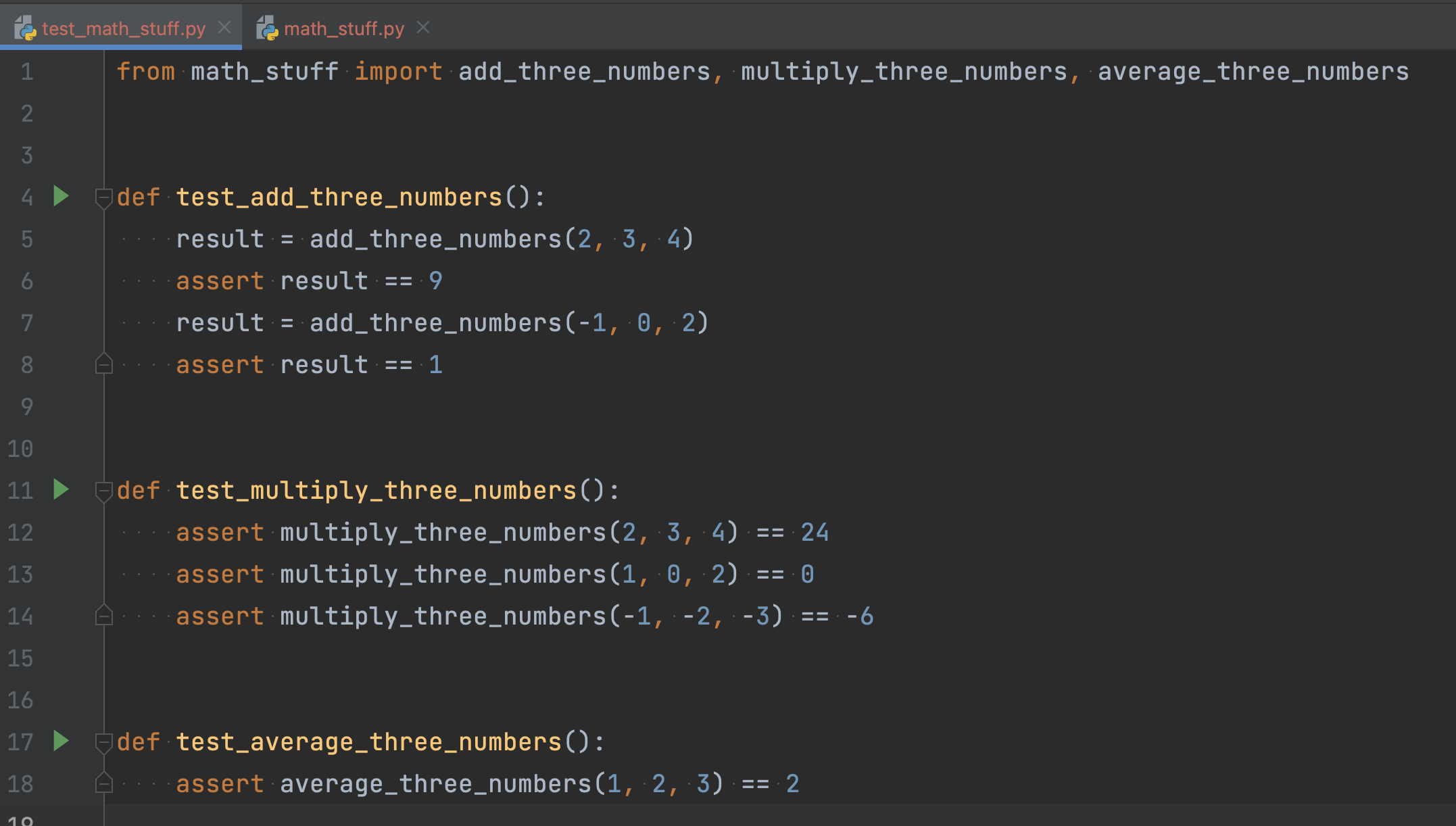You can click each triangle to run the test and see the output in the lower half of PyCharm: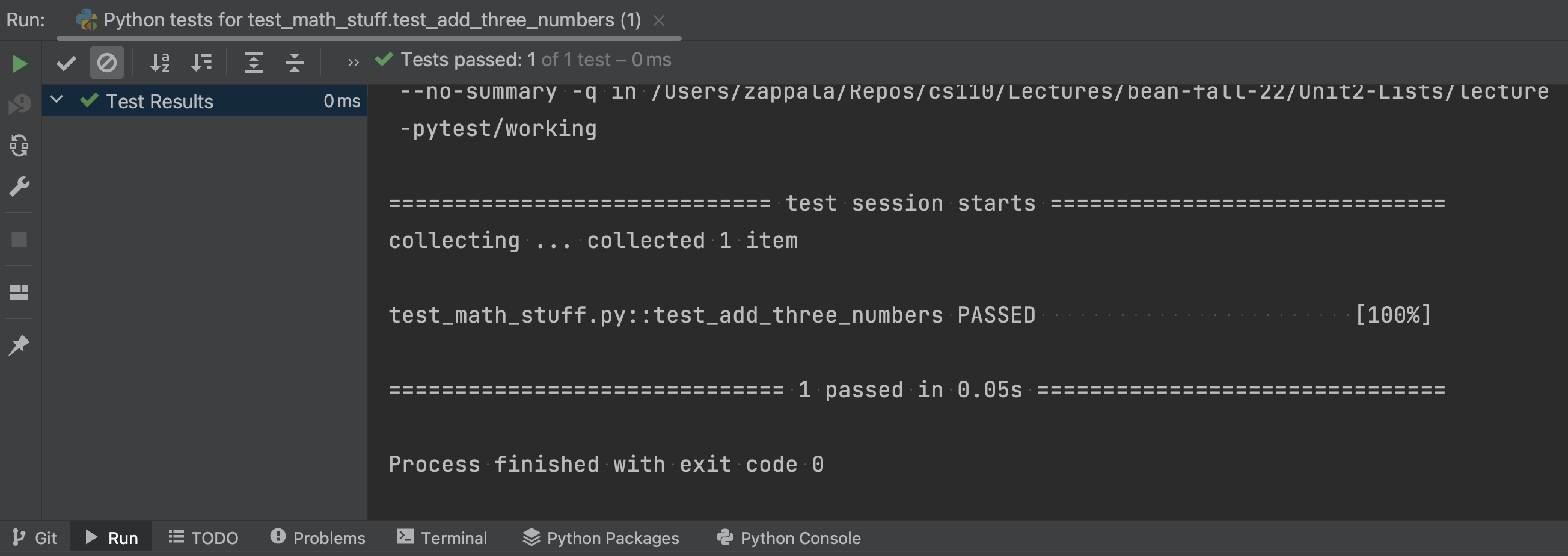If you right-click on the test file and choose Run Python tests for test... then you can run all the tests in the file: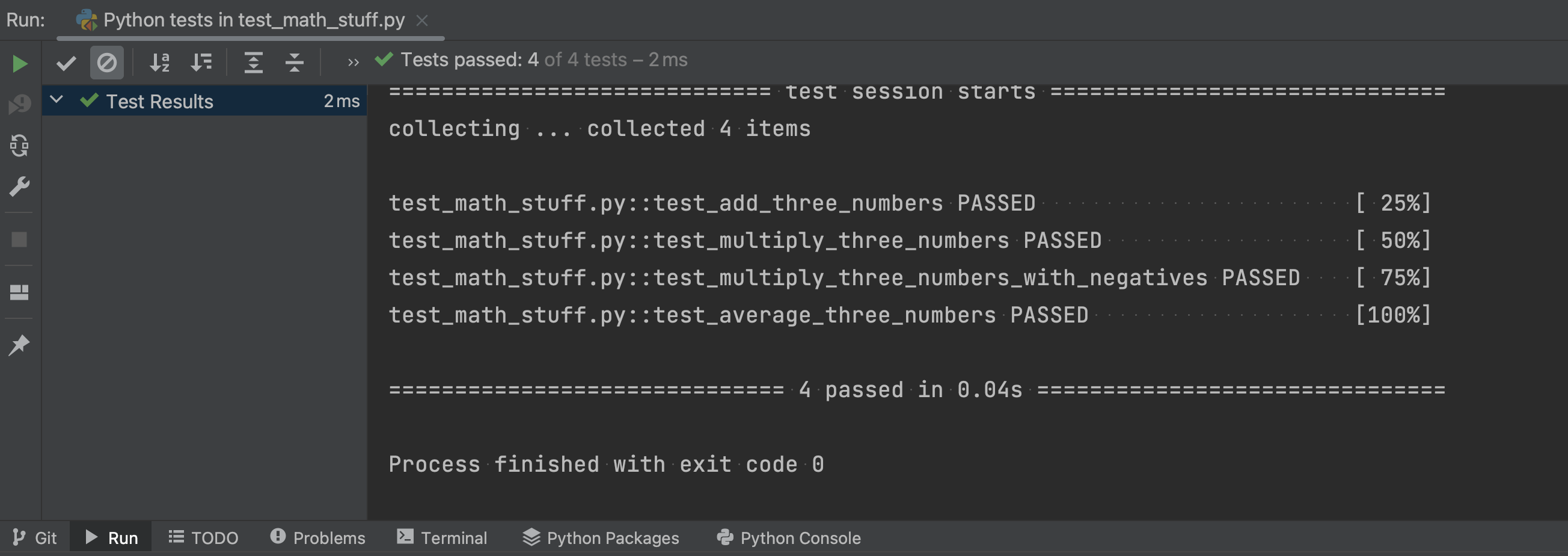## Running tests on the command line

You can also run tests from the command line. First, navigate to the same directory where your test code is located. Then you can run:

pytest test_math_stuff.py

This will run all the tests in that file: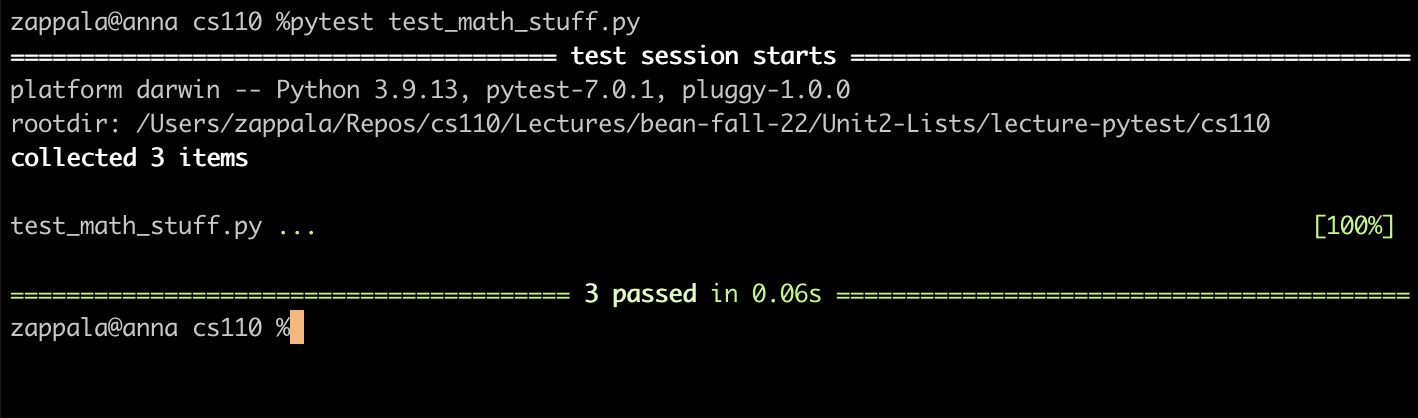If you type just:

pytest

this will run the tests in all test files in that directory or any subdirectories.

## When tests fail

Sometimes, if your code is not correct, you will fail tests. We would like to think this would never happen, but it happens to everyone, including people who have been writing code for years. Finding and fixing mistakes is a normal part of writing programs.

This is what pytest will show you when a test fails: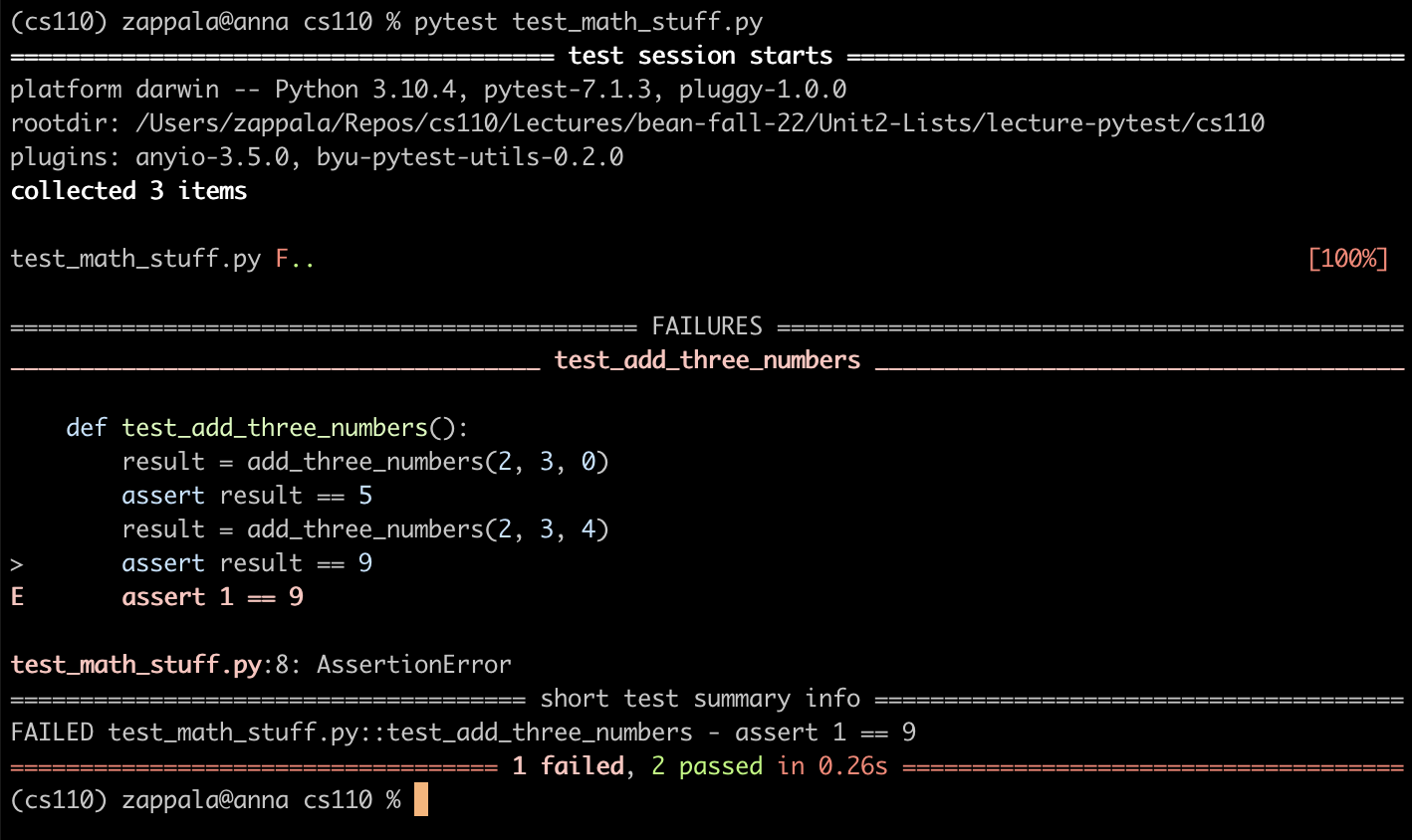Notice that the bottom line shows pytest ran three tests (meaning three functions that start with test_), with 1 failed and 2 passing. The middle of the screen shows the failures, in this case showing test_add_three_numbers() had an error with the second assert. Adding 2, 3, and 4 should return 9, but instead the result was 1.

The E in the left margin shows the error, meaning you tried to assert that 1 is equal to 9. This comes directly from the previous line assert result == 9, so our code returned 1 for the result when calling add_numbers(2, 3, 4).

Once you understand why the test has failed, then you can examine your code. In this case, I had changed the code so that it looked like this:

def add_three_numbers(a, b, c):
return a + b - c

We have tried to add 2, 3, 4, and this returned the result of 2 + 3 - 4, which equals 1. We need to replace the minus sign with a plus sign.

## Debugging with pytest

You can use the PyCharm debugger with pytest when an error fails and the problem in your code is not immediately obvious. Simply put a breakpoint in the test function where the test is failing. You can put a breakpoint right where you know the test is failing: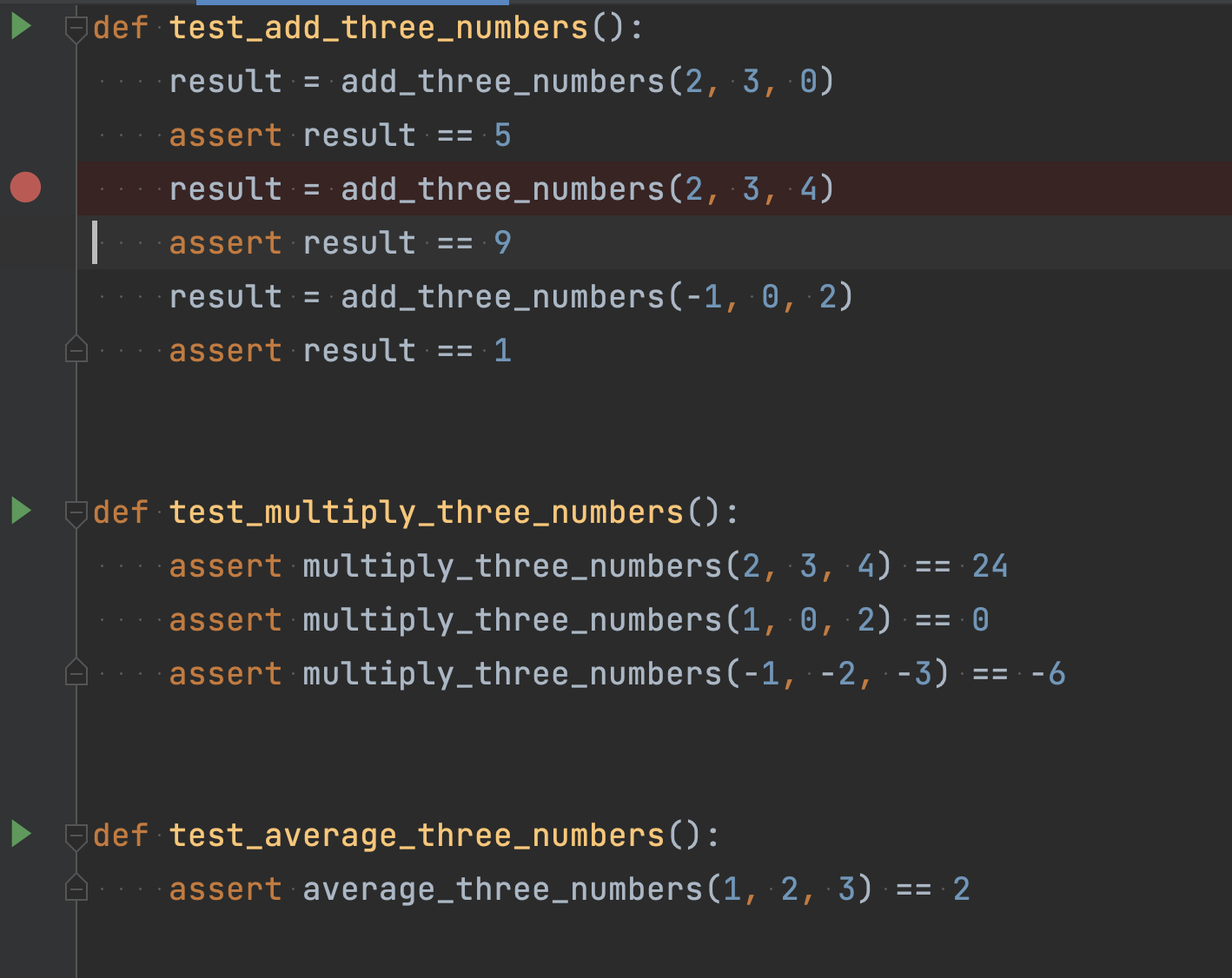You can then use the Step Into button to go into add_three_numbers() for that test and see what is going wrong.